Question

Week 1: Problem 21 Previous Problem List Next (1 point) Definition: The AREA A of the...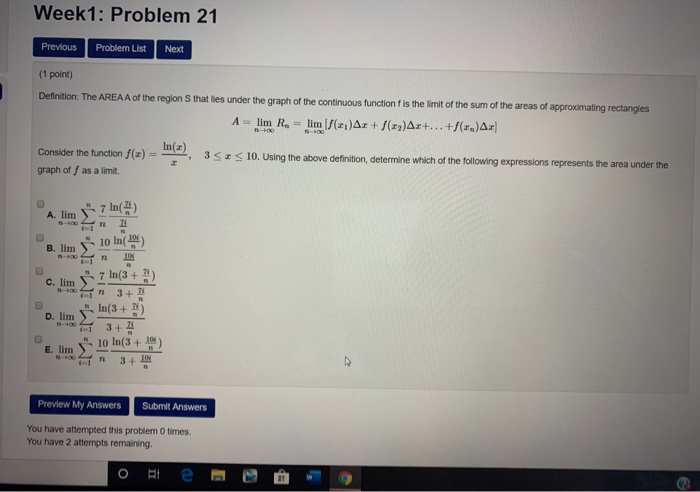Week 1: Problem 21 Previous Problem List Next (1 point) Definition: The AREA A of the region that lies under the graph of the continuous function is the limit of the sum of the areas of approximating rectangles A - lim R. - lim (/(x1)Ar + ()Ar+...+(2.)A: () = 352 10. Using the above definition determine which of the following expressions represents the area under the Consider the function graph off as a limit. A. lim j7 ln() lo in 10 + C. Iim D. lim 7 In(3 + 2) In(3 + 33 3+ 3+ E. Iim 10 In(3 + 0) 3 + Preview My Answers Submit Answers You have attempted this problem 0 times You have 2 attempts remaining. O te w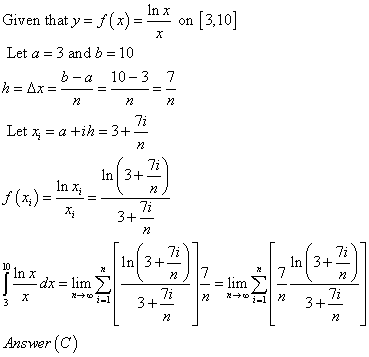Earn Coins

Coins can be redeemed for fabulous gifts.

Similar Homework Help Questions
• ssignment6: Problem 9 Previous Problem Problem List Next Problem (1 point) The area A of the...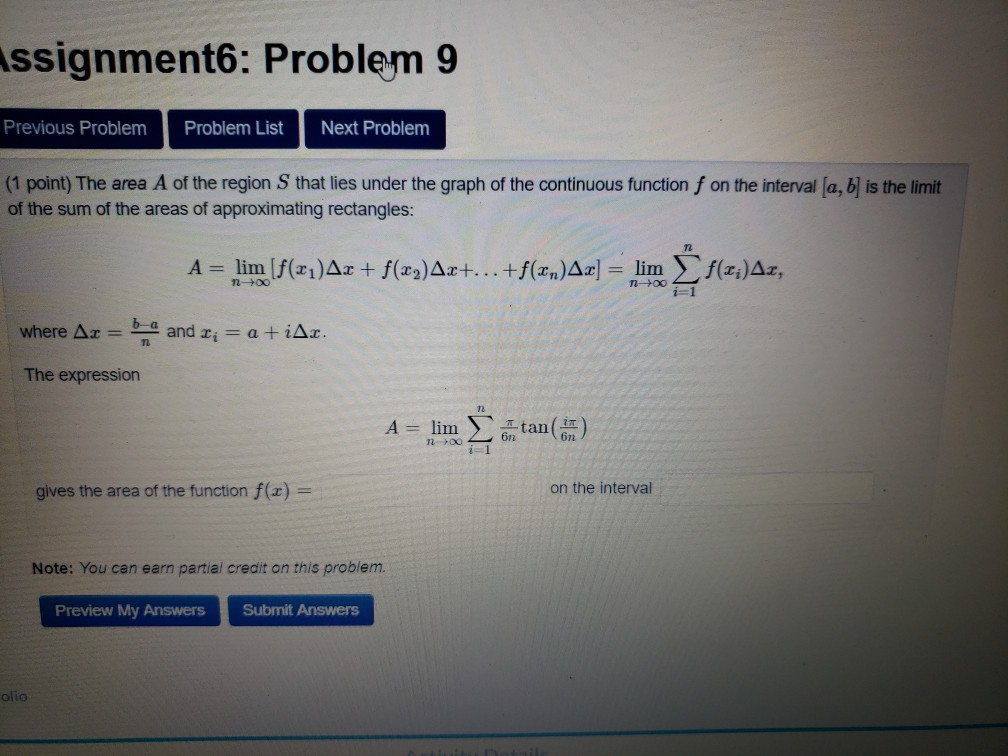ssignment6: Problem 9 Previous Problem Problem List Next Problem (1 point) The area A of the region Sthat lies under the graph of the continuous function f on the interval (a, b) is the limit of the sum of the areas of approximating rectangles: A = lim (f(21)Ar + f(x2)Ax+...+f(xn)Ax] = lim f(x;)Az, n-> ng i=1 where Ax = b and Ti = a +iAr. The expression A = lim Itan(n) 7200 6n2 gives the area of the function f(x)...

• (1 point) Definition: The AREA A of the region that lies under the graph of the...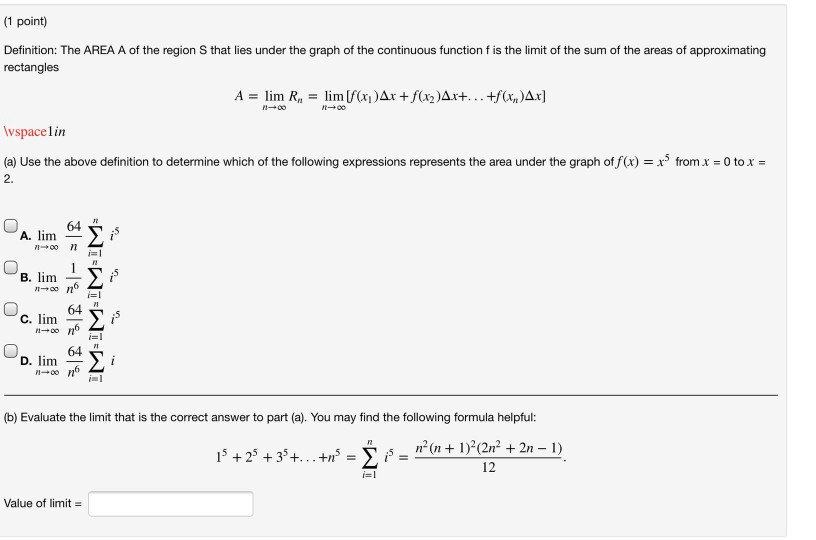(1 point) Definition: The AREA A of the region that lies under the graph of the continuous function f is the limit of the sum of the areas of approximating rectangles A = lim R, = lim [f(x)Ar + f(x2)Ax+... +f(x,y)Ax] 100 Wspacelin (a) Use the above definition to determine which of the following expressions represents the area under the graph of f(x) = x3 from x = 0 to x = 2. 64 A. lim 7100 11 i= B....

• send help for these 4 questions, please show steps Definition: The AREA A of the region...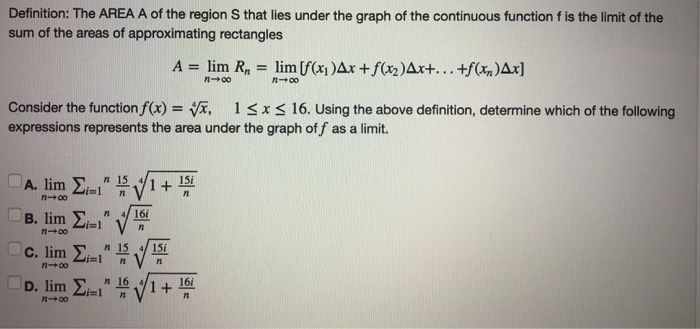send help for these 4 questions, please show steps Definition: The AREA A of the region S that lies under the graph of the continuous function f is the limit of the sum of the areas of approximating rectangles A = lim R, = lim [f(x)Ax +f(x2)Ax+...+f(x)Ax] - 00 Consider the function f(x) = x, 13x < 16. Using the above definition, determine which of the following expressions represents the area under the graph off as a limit. A. lim...

• The area A of the region S that les under the graph of the continuous fun the areas of approximat...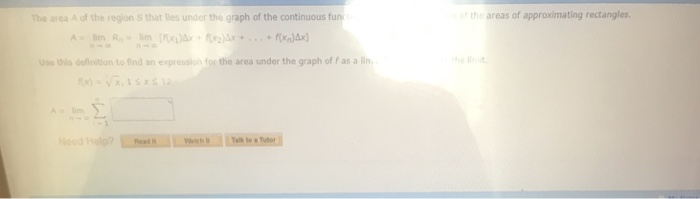The area A of the region S that les under the graph of the continuous fun the areas of approximating rectangles sthis deinition to find an expression for the area under the graph of f as a The area A of the region S that lies under the graph of the continuous function is the limit of the sum of the areas of approximating rectangles Use this definition to find an expression for the area under the graph of f...

• -/2 POINTS SESSCALCET2 5.1.503.XP. The area A of the region that lies under the graph of...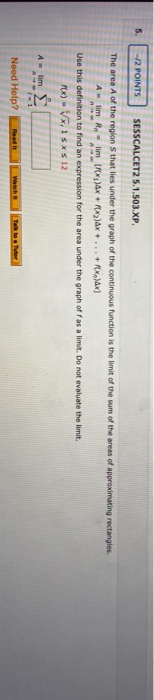-/2 POINTS SESSCALCET2 5.1.503.XP. The area A of the region that lies under the graph of the continuous function is the limit of the sum of the areas of approximating rectangles. lim Rn limf ax + 2)Ax + ... + X)x] Use this definition to find an expression for the area under the graph off as a limit. Do not evaluate the limit. FX) - VX,15* \$ 12 A lim Need Help? Talk to Tutor

• Just need the answer to question 6 using the information provided in the block above question...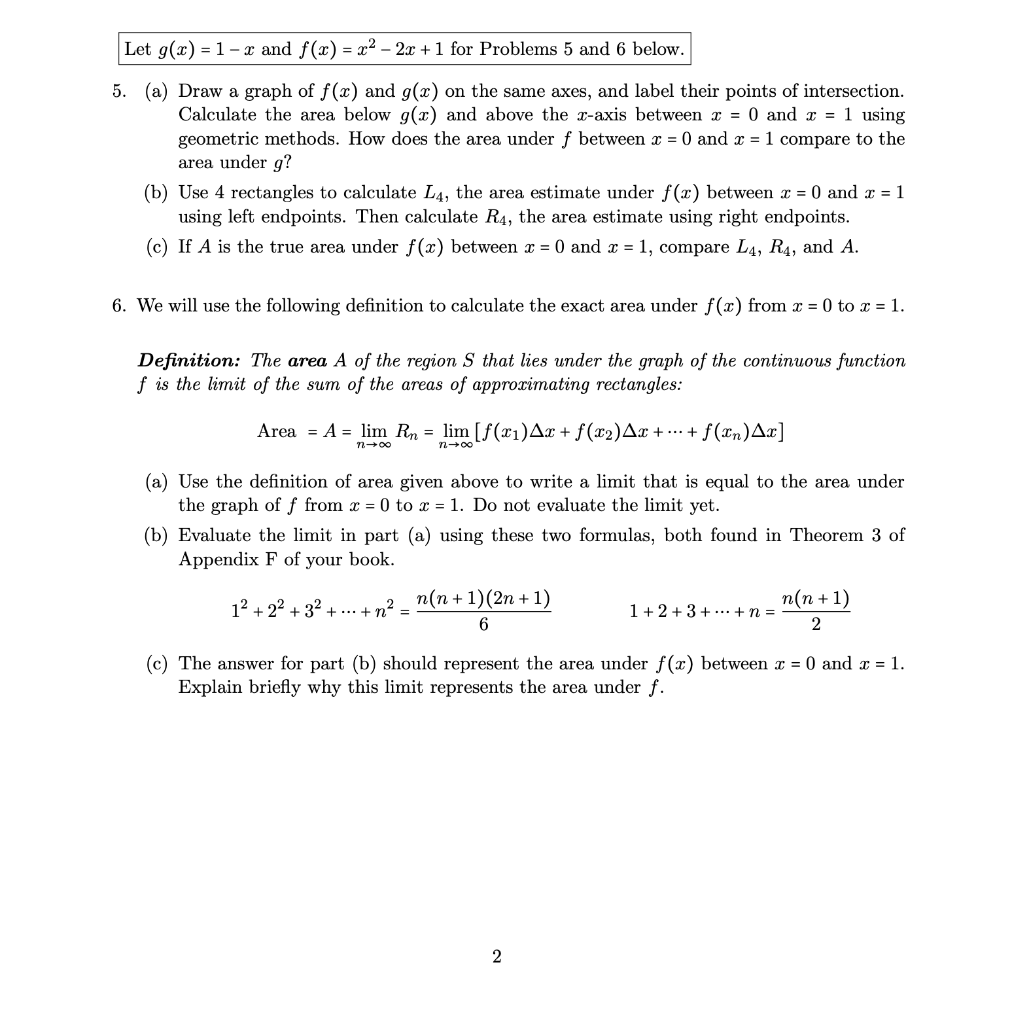Just need the answer to question 6 using the information provided in the block above question 5. Please be clear due to this being a multi-step problem. Thanks    Let g(x) = 1- x and f(x) = x2 - 2x + 1 for Problems 5 and 6 below. 5. (a) Draw a graph of f(x) and g(x) on the same axes, and label their points of intersection. Calculate the area below g(2) and above the z-axis between I = 0 and...

• (2 points) The area A of the region S that lies under the graph of the...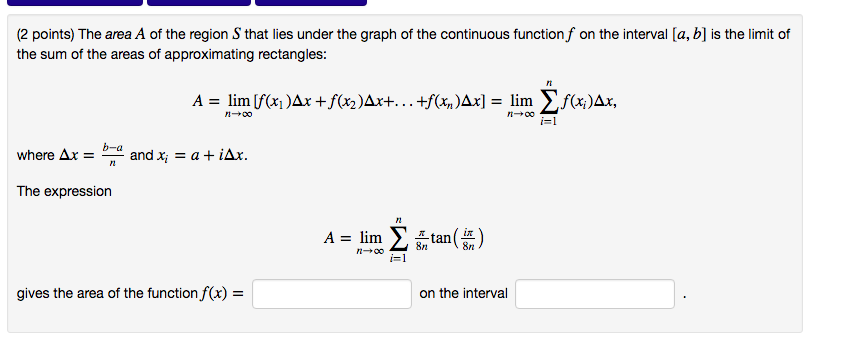(2 points) The area $$A$$ of the region $$S$$ that lies under the graph of the continuous function $$f$$ on the interval $$[a, b]$$ is the limit of the sum of the areas of approximating rectangles:$$A=\lim _{n \rightarrow \infty}\left[f\left(x_{1}\right) \Delta x+f\left(x_{2}\right) \Delta x+\ldots+f\left(x_{n}\right) \Delta x\right]=\lim _{n \rightarrow \infty} \sum_{i=1}^{n} f\left(x_{i}\right) \Delta x$$where $$\Delta x=\frac{b-a}{n}$$ and $$x_{i}=a+i \Delta x$$.The expression$$A=\lim _{n \rightarrow \infty} \sum_{i=1}^{n} \frac{\pi}{8 n} \tan \left(\frac{i \pi}{8 n}\right)$$gives the area of the function $$f(x)=$$ on...

• Previous Problem Problem List Next Problem 3 sin(x) x2 (1 point) Find lim 10 х Enter...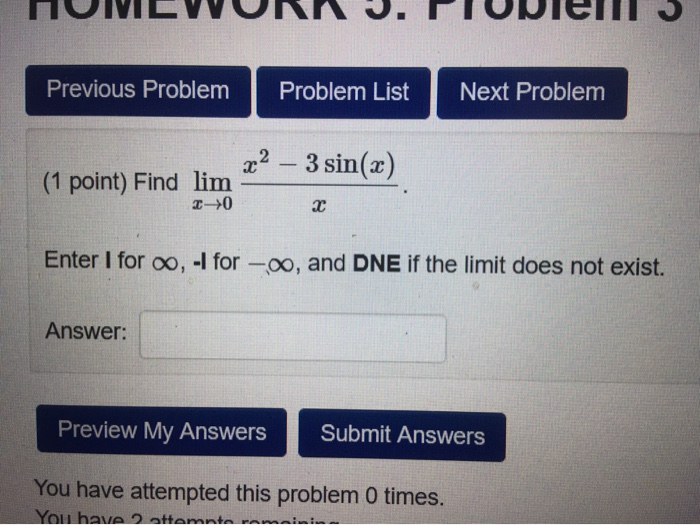Previous Problem Problem List Next Problem 3 sin(x) x2 (1 point) Find lim 10 х Enter I for o, -I for -o, and DNE if the limit does not exist. Answer: Preview My Answers Submit Answers You have attempted this problem 0 times. You have attemate van

• WW10: Problem 13 Previous Problem Problem List Next Problem (1 point) Evaluate the following limit -...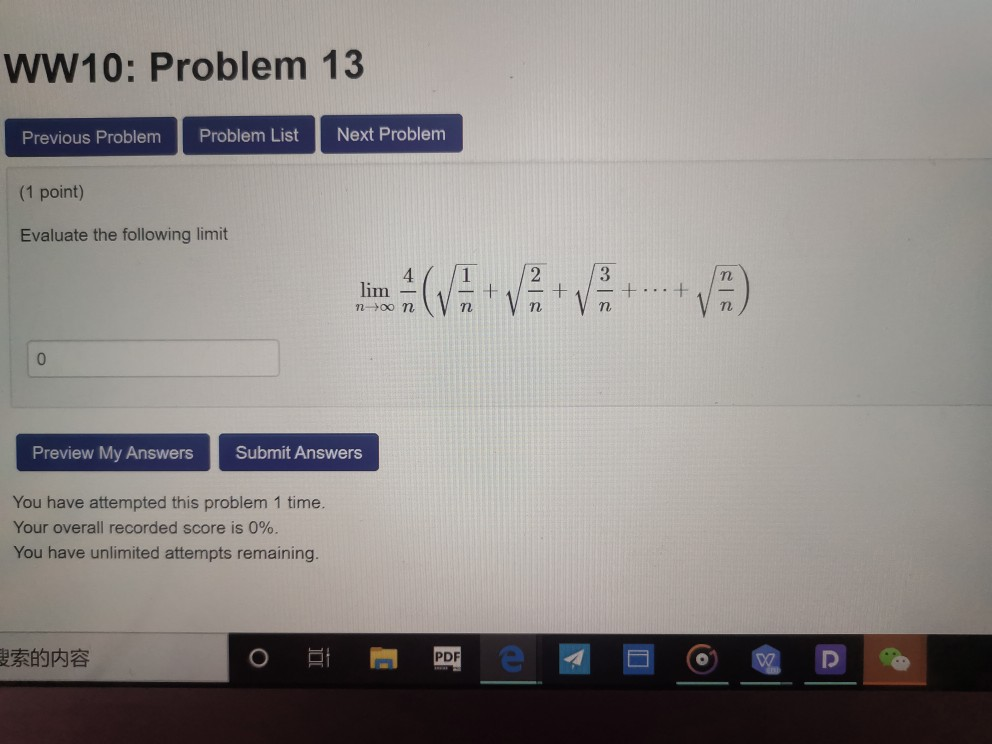WW10: Problem 13 Previous Problem Problem List Next Problem (1 point) Evaluate the following limit - (VA-VV lim 3160 .. . + noon Preview My Answers Submit Answers You have attempted this problem 1 time. Your overall recorded score is 0%. You have unlimited attempts remaining. REAP

• MA441-1.3-Limit-Laws: Problem 1 Previous Problem Problem List Next Problem (1 point) Using: lim f(x) = -3...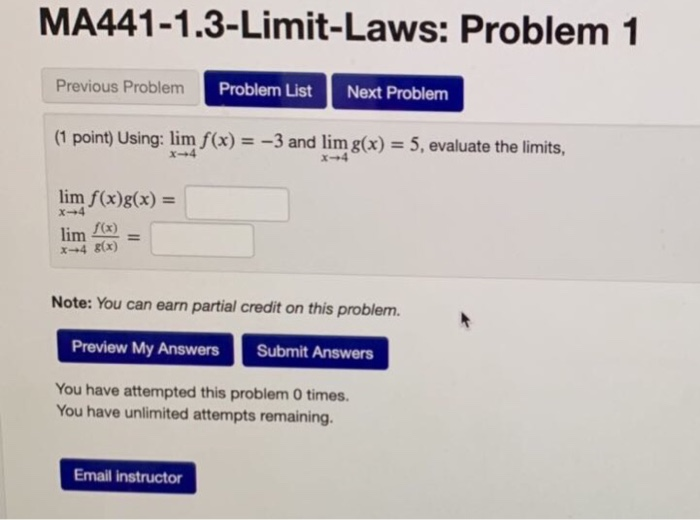MA441-1.3-Limit-Laws: Problem 1 Previous Problem Problem List Next Problem (1 point) Using: lim f(x) = -3 and lim g(x) = 5, evaluate the limits, lim f(x)g(x) = lim f(x) x +4 (x) Note: You can earn partial credit on this problem. Preview My Answers Submit Answers You have attempted this problem 0 times. You have unlimited attempts remaining. Email instructor